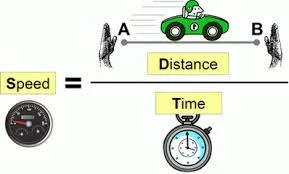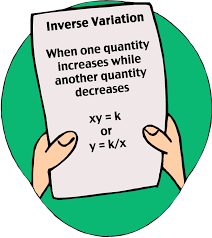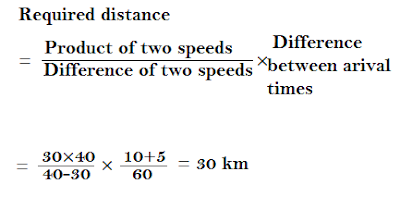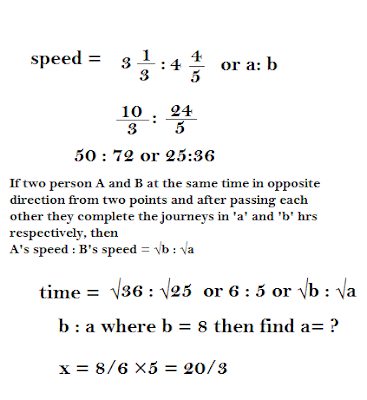New Student User - Use Code HELLO

# Time and Distance - Concepts and TricksIn our daily life, we hear this question where have you been and how much time you have just wasted. Is this much time really needs to go to a certain place and we most probably answered that "It was a huge traffic in there" But what happen if we measure the same time and distance. So today I am going to tell you some things about time and distance not for daily life but for exams.

Speed is always measure in kilo meters per hour(km/hr) and meter per second (m/sec) in our case.To convert speed from km/hr to m/sec.
1 kilo meter = 1000 meter ;
1 hour = 3600 seconds ; Let speed = x.
x km/hr = ( x × 5/18 ) m/sec  [1000/3600 or 5/18]

To convert speed from m/sec to km/hr.
x m/sec = ( x × 18/5 ) km/hr. [3600/1000 or 18/5 ]
Example :
Express a speed of 54 km/hr in meter per second and 15 meter per second into km/hr.
5 km/hr = 54×5/18
5 km/hr = 15 m/sec
Now 15 m/sec = 15 × 18/5
15 m/sec = 54 km/hr.

#### A train runs at the rate of 45 km an hour. What is its speed in meters per second ?

Sol:
45×5/18 = 25/2 = 12.5 m/s
you need here some basic formulas to solve these problems and those are :
(1) Distance = Speed × Time (2) Speed = Distance/Time (3) Time = Distance / Speed

#### A train running with the speed of 90 km/hr. How many meters will it travel in 15 minutes ?

Sol: Speed = 90 km/hr Time = 15 min , Distance = x meters
Now here in this question we have to find out the distance in meters that's why we need to convert the speed in meter per second and also time in seconds.
Speed = 90×5/18 = 25 m/sec and Time = 15×60 = 900 sec
Distance = Speed × Time
Distance = 25×900 = 22500 meters

### 1.Time and Speed inverse to each other#### Walking 2/7 of his usual rate , a boy reaches his school 10 min. late.Find his usual time to reach school ?

Sol:
[ Note : the ratio of speed and time is inverse to each other.like if speed = x:y then time = y:x.
speed is inverse to time. ]
let usual time = x
speed = 2/7 so time = 7/2 because they are inverse to each other.
Difference in time = 10 min
Here you would have seen that now more time is consuming then the previous one or actual time.that's why Difference in time = New Time - Actual Time
extra time = (7/2-1) usual time = 10 minutes
5/2 × usual time = 10
usual time = 4

#### If a man walks to his office at 5/4 of his usual rate, he reaches office 30 min. early than usual. What is his usual time to reach office ?

Sol:
In this question new time is less than the usual time so difference in time = Usual Time - New Time
1-4/5 usual time = 30/60
1/5×x =30/60
x = 5/2
x = 2.5

#### If 'B' is 20% faster than 'A', then what time will he take to travel the distance which 'A' travels in 20 minutes ?

Sol:
Speed = S and Time =T
S1t1 = S2 t2 =Distance
100×S1×t1=120×S2×t2
100×S/120×S × 20 = t2
5/6×20 = t2; t2 = 50/3 ; t2 = 162/3

### 2.Average Speed

• same distance
• distance is not same
1. Same Distance
If a moves at S1 speed for a distance and at S2 speed for the same distance, then
Average speed = 2×S1×S2/S1+S.

#### A man covers a certain distance by car driving at 70 km/hr and he returns back to the starting point riding on a scooter at 55 km/hr. Find his average speed for the whole journey ?

Sol:
Average speed = 2×70×55/70+55 km/hr
= 61.6 km/hr.

2. Distance is not same
Average speed = total distance/total time

#### A car travels 600 km in 11 hours and another 800 km in 17 hours. Find the average speed of the car.

Sol: total distance / total time = 600+800/11+17= 50 km/hr

#### A bus moves 300 km at a speed of 45 km/hr and then it increases its speed to 60 km/hr to travel another 500 km. Find the average speed of the bus.

Sol : Avg Speed = total distance/total time
total distance = 300+500=800
total time = t1+t2
= (distance / speed1)+(distance / speed2) = 300/45 + 500/60 = 45/3 hr
Avg speed = total distance /total time
A.S = 800/45/3
= 531/3 km/hr

### Distance covered is Same

#### A man covers a certain distance between his house and office on scooter. Having an average speed of 30 km/hr, he is late by 10 min. However with a speed of 40 km/hr, he reaches  his office 5 min earlier. Find the distance between his house and office.

Sol: Let the distance be x km.

Time taken to cover x km at 30 km/hr = x/30 hrs.

Time taken to cover x km at 40 km/hr = x/40 hrs.

Difference between the time taken = 15 min = 1/4 hr.
x/30 x/40 = 1/4 or 4x - 3x = 30 or x = 30
Hence, the required distance is 30 km.#### The distance between two station, Delhi and Amritsar, is 450 km. A train starts at 4 p.m. from Delhi and moves towards Amritsar  at an average speed of 60 km/hr. Another train starts from Amritsar at 3.20 p.m. and moves towards Delhi at an average speed of 80 km/hr. how far from Delhi will the two trains meet and at what time ?

Sol: Suppose the trains meet at a distance of x km from Delhi. Let the trains from Delhi and Amritsar be A and B respectively ,Then [ Time taken by B to cover (450-x) km ] - [Time taken by A to cover x km ] = 40/60
450-x/80 - x/60 = 40/60
x = 170
Thus the train meets at a distance of 170 km from Delhi. Time taken by A to cover 170 km = (170/60) hrs = 2 hrs 50 min
So, the trains meet at 6.50 p.m.

### Distance covered is not Same

#### A motor car does a journey in 10 hours, the first half at 21 km/hr and the second half at 24 km/hr. Find the distance.

Sol:### Running in Same Direction

#### Two train for Patna leave Delhi at 10 a.m. and 10:30 a.m. and travel at 60 km/hr 75 km/hr respectively. How many kilometers from Delhi will the two trains be together ?

Sol:
Meeting point's distance = 60 × 75 / 75-60  × 30/60
= 150 km

#### Two runners cover the same distance at the rate of 15 km and 16 km per hour respectively. Find the distance travelled when one takes 16 minutes longer than the other.

Sol:
16×15/16-15 × 16/60

#### A carriage driving in a fog passed a man who awas walking at the of 3 km an hour in the same direction. He could see the carriage for 4 minutes and it was visible to him upto a distance of 100 meter. What was the speed of the carriage ?### Running in opposite DirectionIf two person A and B at the same time in opposite direction from two points and after passing each other they complete the journeys in 'a' and 'b' hrs respectively, then
A's speed : B's speed = √b : √a

#### A man sets out to cycle from Delhi to Rohtak and at the same time another man starts from Rohtak to cycle to Delhi. After passing another man starts from Rohtak to cycle to Delhi. After passing each other they complete their journeys in 31/3 and 44/5 hours respectively. At what rate does the second man cycle if the first cycles at 8 km per hour ?

Sol:
Speed = 31/3 : 44/5 or 10/3 : 24/5 or 25:36
Time = √b : √a or √36:√25 or 6 : 5
8/6 × 5 = 20/3 or 62/3#### The distance between two station, Delhi and Amritsar, is 450 km. A train starts at 4 p.m. from Delhi and moves towards Amritsar  at an average speed of 60 km/hr. Another train starts from Amritsar at 3.20 p.m. and moves towards Delhi at an average speed of 80 km/hr. how far from Delhi will the two trains meet and at what time ?

Sol: Suppose the trains meet at a distance of x km from Delhi. Let the trains from Delhi and Amritsar be A and B respectively ,Then
[Time taken by B to cover (450-x) km ] - [Time taken by A to cover x km ] = 40/60
450-x/80 - x/60 = 40/60 ; x = 170.
Thus the train meets at a distance of 170 km from Delhi. Time taken by A to cover 170 km = (170/60) hrs = 2 hrs 50 min.So, the trains meet at 6.50 p.m.